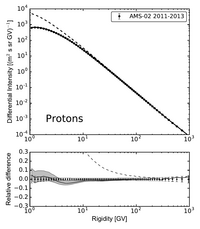HelMod-4 The Heliospheric Modulation Model Online Calculator Version TestHelmod-4 Version 1.0

In HelMod v4.0.0 Online version is aligned with those in Boschini et al 2019. The actual best configuration that reproduce both high and low activity period is: $$\rho_i=0.065$$, $$g_{low}=0.5$$,  $$\delta_{m}=2\times 10^{-5}$$, $$K_{0,HS}=3\times 10^{-5}$$.

Helmod-3 Version 4.0

In HelMod v3.4.0 Online version is aligned with those in Boschini et al 2017. The actual best configuration that reproduce both high and low activity period is: $$\rho_i=0.060$$, $$g_{low}=0.3$$,  $$\delta_{m}=2\times 10^{-5}$$.

Helmod-3 Version 1.0

In HelMod v3.1.0 the default LIS is obtained by GALPROP toughether with HelMod as described in LIS page. The actual best configuration that reproduce both high and low activity period is: $$\rho_i=0.058$$, $$g_{low}=0.2$$,  $$\delta_{m}=4\times 10^{-5}$$.

Helmod-3 Version 0.1

In HelMod v3.0.1 script accounts for asymmetric errors. The actual best configuration that reproduce both high and low activity period is: $$\rho_i=0.062$$, $$g_{low}=0.1$$,  $$\delta_{m}=4\times 10^{-5}$$.

Helmod-3 Version 0.0

In HelMod v3.0.0 parameters were tuned to minimize discrepancies of modulated spectra from Experimental data from High to Low activity period. The procedure was presented in Della Torre et al 2016a and  Della Torre et al 2016b. The actual best configuration that reproduce both high and low activity period is: $$\rho_i=0.06$$, $$g_{low}=0.2$$,  $$\delta_{m}=4\times 10^{-5}$$.The effective heliosphere is divided in 15 regions from 0 to 100 AU. Each i-th region is filled with heliosphere parameters evaluated for i carrington rotations before the target period. The heliosphere parameters are evaluated from Omniweb, Silso and WSO.

#### Bibliography

 M J Boschini, S Della Torre, M Gervasi, G La Vacca and P G Rancoita. The HelMod Model in the Works for Inner and Outer Heliosphere: from AMS to Voyager Probes Observations.  Advances in Space Research 64(12):2459 - 2476, 2019. M J Boschini, S Della Torre, M Gervasi, G La Vacca and P G Rancoita.  Propagation of Cosmic Rays in Heliosphere: the HelMod Model.  Adv. Space Res. 62(10):2859 - 2879, 2018. S Della Torre, M Gervasi, D Grandi, G Johannesson, G La Vacca, N Masi, I V Moskalenko, E Orlando, T A Porter, L Quadrani, P G Rancoita and D Rozza. (2016a) From Observations near the Earth to the Local Interstellar Spectra, Proceedings of the 25th European Cosmic Rays Symposyum (ECRS2016), Septembers 4-9,2016 (Torino, Italy) . S Della Torre, M Gervasi, D Grandi, G Johannesson, G La Vacca, N Masi, I V Moskalenko, E Orlando, T A Porter, L Quadrani, P G Rancoita and D Rozza. (2016b) HelMod: a Comprehensive Treatment of the Cosmic Ray transport through the Heliosphere, Proceedings of the 25th European Cosmic Rays Symposyum (ECRS2016), Septembers 4-9,2016 (Torino, Italy)